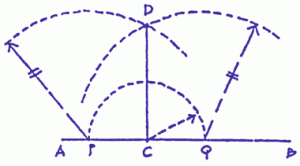## The Library of Practical Geometry

Practical help to solve today’s complex design problems.

# Take a Tour (A)

### To draw a straight line perpendicular to a given straight line from any point on it.This method of drawing a perpendicular is one of the earliest constructions of Practical Geometry known. The ancient Egyptians and Greeks used it extensively. The ability to construct an accurate perpendicular line or right angle at a specific point on a line is critical in any actual lay-out of a square corner or a square grid pattern. It is definitely necessary when using a small drawing triangle or a builder’s square is not practical.

• Given a straight line AB and point C on it.
• With point C as centre, set compass to any radius (in this case CQ) and draw a semi-circle around the centre.
• With point P as centre, draw arc radius equal to PQ.
• With point Q as centre, draw arc radius equal to QP. The arcs about point P and Q will intersect at point D.
• Connect point C to point D and the line CD will be the desired perpendicular.

Carpenters, tile setters, and landscapers among others, will find this ancient method extremely useful. Understanding this basic method of construction is critical for ongoing application of the constructions of Practical Geometry.

NEXT PAGE OF TOUR >